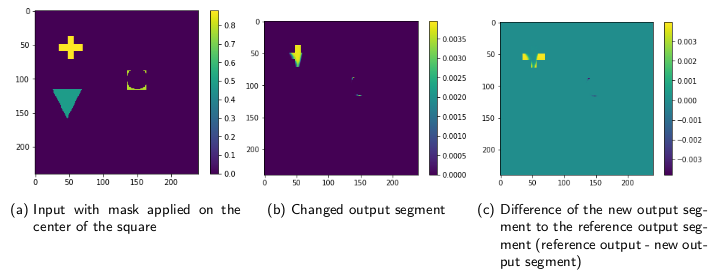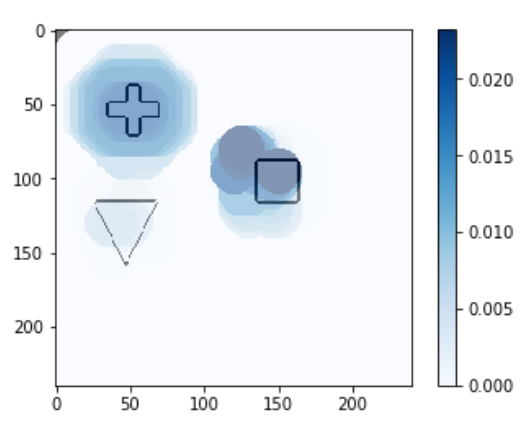## Introduction¶

Hausdorff Distance Masks is a new method developed for the interpretability of image segmentation models. Like RISE, it is a black box method. The output of the method has a higher resolution than RISE and is more accurate.

## How does it work?¶

The first part of the algorithm is the occlusion of parts of the input image. We iterate over the image in a linear fashion, from left to right and from top to bottom, based on a pixel offset between every row and column defined as a parameter of the algorithm. For every position that is encountered, we create a new image. On this image, we draw a filled black circle at the specific position.

The images with the masks applied from above are then passed through the neural networks. The output segmentation may not change or only change slightly when the mask occludes an unimportant part of the image. Applying the mask on important parts of the image can change the segmentation output significantly.Applying the mask on center of the square (a) significantly changes the segment output (b) of the neural network. The network even includes a part of the square in the output segment.

To asses how big the change of the segmentation output is, we use the Hausdorff distance function between the new segmentation and the ground truth.

To visualize all the distances from the output of the masked image, a new blank image with the same size as the input image is generated. Next, we iterate over all the positions where masks have been applied to the input image. Each position has an associated Hausdorff distance which represents the distance of the output segment generated by the masked image and the ground truth segment. At each position, we draw a circle with the same diameter as used when generating the mask. The color used to fill this circle represents the Hausdorff distance between the output segment generated by placing a circle at this exact position and the ground truth segment. The color map is scaled to the minimum and maximum Hausdorff distance encountered on all positions.Visualization of all Hausdorff distances corresponding to a mask at the same position. Intensity of the circle color is based on the Hausdorff distance at this position. The input image was processed with the canny edge detector.

## Example¶

```from interpret_segmentation import hdm
import torch
import matplotlib.pyplot as plt

device = torch.device("cuda:0" if torch.cuda.is_available() else "cpu")

# a PyTorch model
model = ...

# a PyTorch dataset
dataset = ...

# ground truth segment (PyTorch 2D tensor)
segment = ...

# input image  (PyTorch 2D tensor)
image = ...

# initialize the explainer with image width and height

# apply masks and calculate distances
result = explainer.explain(model, image, segment, device)

# generate circle map visualizations
raw = result.circle_map(hdm.RAW, color_map='Blues')
better = result.circle_map(hdm.BETTER_ONLY, color_map='Greens')
worse = result.circle_map(hdm.WORSE_ONLY, color_map='Reds')

# show with matplotlib...
plt.imshow(raw)
plt.show()

# ...or save to disk
raw.save('raw.png')
```

## Class documentation¶

class `interpret_segmentation.hdm.``HausdorffDistanceMasks`(image_width, image_height)

`__init__`(image_width, image_height)

Initialize the explainer.

Parameters: image_width – Input image width image_height – Input image height
`generate_masks`(circle_size, offset, normalize=False)

Generate the masks for the explainer. A circle_size of 15 pixels and an offset of 5 pixel work good on a 240x240 image.

Parameters: circle_size – Diameter in pixels of the circles drawn onto the image offset – The distance in pixel between every drawn circle normalize – Normalize generated masks to mean 0.5
`explain`(model, image, segment, device, channel=-1)

Explain a single instance with Hausdorff Distance masks. The model needs to reside on the device given as a parameter to this method.

Returns a `HDMResult` instance.

Parameters: model – A PyTorch module image – The input image to be explained (2D PyTorch tensor or numpy array) segment – The ground truth segment (2D PyTorch tensor or numpy array) device – A PyTorch device channel – Channel on which the mask should be applied, -1 for all channels (default) An instance of `HDMResult`
`apply_mask`(image, mask)

Apply a mask on an image. By default, this does a `torch.min(image, mask)`, but can be overwritten to do something else.

Parameters: image – The input image, 2D numpy array mask – The mask, 2D numpy array
`calculate_distance`(output, segment)

Calculate the difference between the network output and the ground truth segment. Default implementation is the Hausdorff distance, but this can be replaced by any other distance function.

Parameters: output – Neural network output, 2D numpy array segment – Ground truth segment, 2D numpy array A number representing the distance between output and segment
class `interpret_segmentation.hdm.``HDMResult`(distances, baseline, image_width, image_height, circle_size, offset)

Result class for the Hausdorff Distance masks algorithm. Instanced by HausdorffDistanceMasks class.

`distances`(result_type)

Returns distances as a 2D matrix. Every matrix entry corresponds to one applied mask.

• hdm.RAW: The raw Hausdorff Distance
• hdm.BETTER_ONLY: Only distances where the occlusion by the mask increased the accuracy of the output.
• hdm.WORSE_ONLY: Only distances where the occlusion by the mask decreased the accuracy of the output.
Parameters: result_type – hdm.RAW, hdm.BETTER_ONLY, hdm.WORSE_ONLY numpy 2D matrix
`circle_map`(result_type, color_map='Reds')

Generates the Hausdorff Distance Mask visualization.

• hdm.RAW: The raw Hausdorff Distance
• hdm.BETTER_ONLY: Only distances where the occlusion by the mask increased the accuracy of the output.
• hdm.WORSE_ONLY: Only distances where the occlusion by the mask decreased the accuracy of the output.
Parameters: result_type – hdm.RAW, hdm.BETTER_ONLY, hdm.WORSE_ONLY color_map – A matplotlib color map PIL image